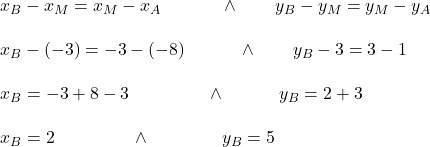## Point M is the midpoint of AB. The coordinates of point A are (-8, 1) and the coordinates of M are (-3,3). What are the coordinates of

Question

Point M is the midpoint of AB. The coordinates of point A are (-8, 1) and the coordinates of M are (-3,3).
What are the coordinates of point B?
The coordinates of point B are

in progress 0
5 months 2021-08-27T12:25:03+00:00 1 Answers 3 views 0